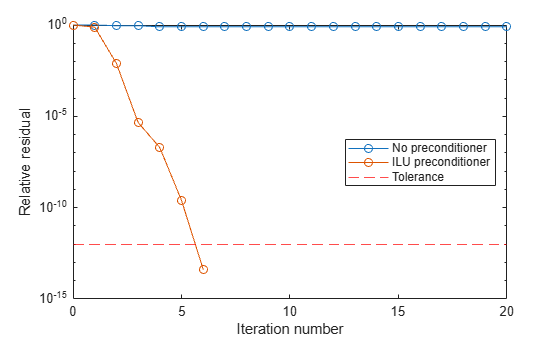Documentation

# qmr

Quasi-minimal residual method

## Syntax

```x = qmr(A,b) qmr(A,b,tol) qmr(A,b,tol,maxit) qmr(A,b,tol,maxit,M) qmr(A,b,tol,maxit,M1,M2) qmr(A,b,tol,maxit,M1,M2,x0) [x,flag] = qmr(A,b,...) [x,flag,relres] = qmr(A,b,...) [x,flag,relres,iter] = qmr(A,b,...) [x,flag,relres,iter,resvec] = qmr(A,b,...) ```

## Description

`x = qmr(A,b)` attempts to solve the system of linear equations `A*x=b` for `x`. The `n`-by-`n` coefficient matrix `A` must be square and should be large and sparse. The column vector `b` must have length `n`. You can specify `A` as a function handle, `afun`, such that `afun(x,'notransp')` returns `A*x` and `afun(x,'transp')` returns `A'*x`.

Parameterizing Functions explains how to provide additional parameters to the function `afun`, as well as the preconditioner function `mfun` described below, if necessary.

If `qmr` converges, a message to that effect is displayed. If `qmr` fails to converge after the maximum number of iterations or halts for any reason, a warning message is printed displaying the relative residual `norm(b-A*x)/norm(b)` and the iteration number at which the method stopped or failed.

`qmr(A,b,tol)` specifies the tolerance of the method. If `tol` is `[]`, then `qmr` uses the default, `1e-6`.

`qmr(A,b,tol,maxit)` specifies the maximum number of iterations. If `maxit` is `[]`, then `qmr` uses the default, `min(n,20)`.

`qmr(A,b,tol,maxit,M)` and `qmr(A,b,tol,maxit,M1,M2)` use preconditioners `M` or `M = M1*M2` and effectively solve the system `inv(M)*A*x = inv(M)*b` for `x`. If `M` is `[]` then `qmr` applies no preconditioner. `M` can be a function handle `mfun` such that `mfun(x,'notransp')` returns `M\x` and `mfun(x,'transp')` returns `M'\x`.

`qmr(A,b,tol,maxit,M1,M2,x0)` specifies the initial guess. If `x0` is `[]`, then `qmr` uses the default, an all zero vector.

`[x,flag] = qmr(A,b,...)` also returns a convergence flag.

Flag

Convergence

`0`

`qmr` converged to the desired tolerance `tol` within `maxit `iterations.

`1`

`qmr` iterated `maxit` times but did not converge.

`2`

Preconditioner `M` was ill-conditioned.

`3`

The method stagnated. (Two consecutive iterates were the same.)

`4`

One of the scalar quantities calculated during `qmr` became too small or too large to continue computing.

Whenever `flag` is not `0`, the solution `x` returned is that with minimal norm residual computed over all the iterations. No messages are displayed if the `flag` output is specified.

`[x,flag,relres] = qmr(A,b,...)` also returns the relative residual `norm(b-A*x)/norm(b)`. If `flag` is `0`, ```relres <= tol```.

`[x,flag,relres,iter] = qmr(A,b,...)` also returns the iteration number at which `x` was computed, where `0 <= iter <= maxit`.

`[x,flag,relres,iter,resvec] = qmr(A,b,...)` also returns a vector of the residual norms at each iteration, including `norm(b-A*x0)`.

## Examples

### Using qmr with a Matrix Input

This example shows how to use `qmr` with a matrix input. The code:

```n = 100; on = ones(n,1); A = spdiags([-2*on 4*on -on],-1:1,n,n); b = sum(A,2); tol = 1e-8; maxit = 15; M1 = spdiags([on/(-2) on],-1:0,n,n); M2 = spdiags([4*on -on],0:1,n,n); x = qmr(A,b,tol,maxit,M1,M2);```

displays the message:

```qmr converged at iteration 9 to a solution... with relative residual 5.6e-009```

### Using qmr with a Function Handle

This example replaces the matrix `A` in the previous example with a handle to a matrix-vector product function `afun`. The example is contained in a file `run_qmr` that

• Calls `qmr` with the function handle `@afun` as its first argument.

• Contains `afun` as a nested function, so that all variables in `run_qmr` are available to `afun`.

The following shows the code for `run_qmr`:

```function x1 = run_qmr n = 100; on = ones(n,1); A = spdiags([-2*on 4*on -on],-1:1,n,n); b = sum(A,2); tol = 1e-8; maxit = 15; M1 = spdiags([on/(-2) on],-1:0,n,n); M2 = spdiags([4*on -on],0:1,n,n); x1 = qmr(@afun,b,tol,maxit,M1,M2); function y = afun(x,transp_flag) if strcmp(transp_flag,'transp') % y = A'*x y = 4 * x; y(1:n-1) = y(1:n-1) - 2 * x(2:n); y(2:n) = y(2:n) - x(1:n-1); elseif strcmp(transp_flag,'notransp') % y = A*x y = 4 * x; y(2:n) = y(2:n) - 2 * x(1:n-1); y(1:n-1) = y(1:n-1) - x(2:n); end end end```

When you enter

`x1=run_qmr;`

MATLAB® software displays the message

```qmr converged at iteration 9 to a solution with relative residual 5.6e-009```

### Using qmr with a Preconditioner

This example demonstrates the use of a preconditioner.

Load `A = west0479`, a real 479-by-479 nonsymmetric sparse matrix.

```load west0479; A = west0479;```

Define `b` so that the true solution is a vector of all ones.

`b = full(sum(A,2));`

Set the tolerance and maximum number of iterations.

```tol = 1e-12; maxit = 20;```

Use `qmr` to find a solution at the requested tolerance and number of iterations.

`[x0,fl0,rr0,it0,rv0] = qmr(A,b,tol,maxit);`

`fl0` is 1 because `qmr` does not converge to the requested tolerance `1e-12` within the requested 20 iterations. The seventeenth iterate is the best approximate solution and is the one returned as indicated by `it0 = 17`. MATLAB stores the residual history in `rv0`.

Plot the behavior of `qmr`.

```semilogy(0:maxit,rv0/norm(b),'-o'); xlabel('Iteration number'); ylabel('Relative residual');```The plot shows that the solution does not converge. You can use a preconditioner to improve the outcome.

Create the preconditioner with `ilu`, since the matrix `A` is nonsymmetric.

```[L,U] = ilu(A,struct('type','ilutp','droptol',1e-5)); ```
```Error using ilu There is a pivot equal to zero. Consider decreasing the drop tolerance or consider using the 'udiag' option. ```

MATLAB cannot construct the incomplete LU as it would result in a singular factor, which is useless as a preconditioner.

You can try again with a reduced drop tolerance, as indicated by the error message.

```[L,U] = ilu(A,struct('type','ilutp','droptol',1e-6)); [x1,fl1,rr1,it1,rv1] = qmr(A,b,tol,maxit,L,U);```

`fl1` is 0 because `qmr` drives the relative residual to `4.1410e-014` (the value of `rr1`). The relative residual is less than the prescribed tolerance of `1e-12` at the sixth iteration (the value of `it1`) when preconditioned by the incomplete LU factorization with a drop tolerance of `1e-6`. The output `rv1(1)` is `norm(b)`, and the output `rv1(7)` is `norm(b-A*x2)`.

You can follow the progress of `qmr` by plotting the relative residuals at each iteration starting from the initial estimate (iterate number 0).

```semilogy(0:it1,rv1/norm(b),'-o'); xlabel('Iteration number'); ylabel('Relative residual');```## References

 Barrett, R., M. Berry, T. F. Chan, et al., Templates for the Solution of Linear Systems: Building Blocks for Iterative Methods, SIAM, Philadelphia, 1994.

 Freund, Roland W. and Nöel M. Nachtigal, “QMR: A quasi-minimal residual method for non-Hermitian linear systems,” SIAM Journal: Numer. Math. 60, 1991, pp. 315–339.